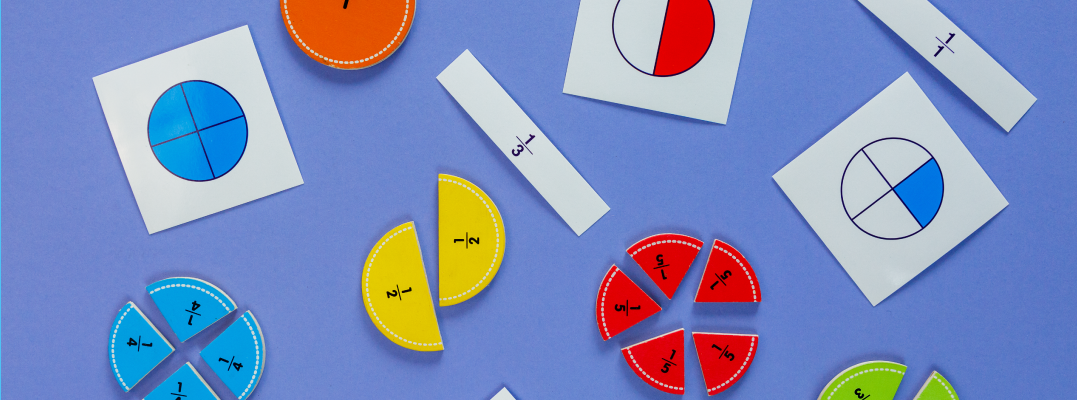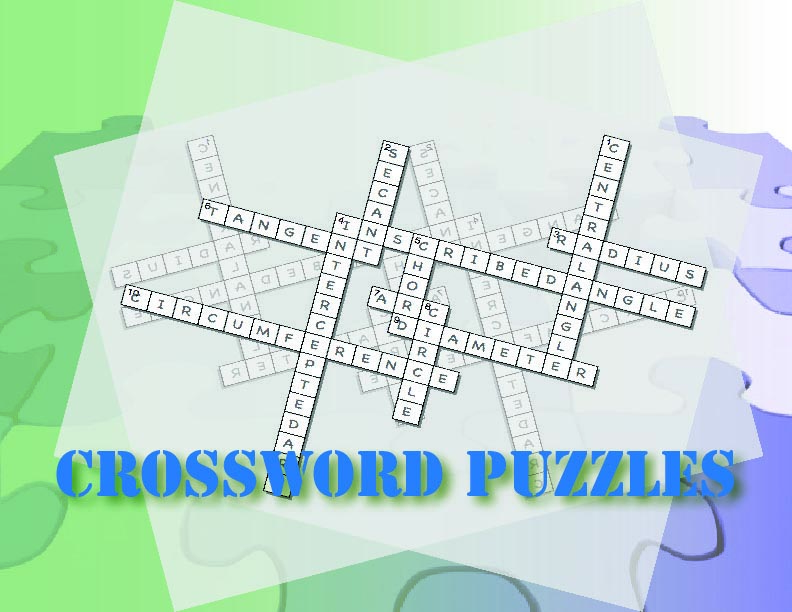# Unit: Comparing and Ordering Fractions### Key topics include:

• Generating equivalent fractions
• Comparing fractions using various strategies
• Ordering fractions using various strategies

### The suggested timeframe for each lesson can be adapted to suit your needs.### Days 1-2: Speaking Math

Introduction of key vocabulary around fractions. This is a gamified experience with word puzzles and other games.

Introduce these terms and definitions

• Fraction
• Numerator
• Denominator
• Equivalent Fraction
• Benchmark Fraction
• Greater Than
• Less Than
• Factor
• Comparing Fractions
• Ordering Fractions
• Unit Fraction

Media4Math Resources:

Goal: A low-stress intro to fractions vocabulary relating to comparing fractions. These two days lay the groundwork of vocabulary and basic concepts. Students compare unit fractions as an introduction to comparing and ordering fractions.### Days 3-4: Building Blocks

A brief review of equivalent fractions as a means of comparing fractions. Students are also shown how to compare fractions indirectly by comparing them to benchmark fractions.

Media4Math Resources:

Goal: To review equivalent fractions and develop a basic understanding of fraction comparisons.### Days 5-6: Putting it Together

Students learn how to compare and order fractions using equivalent fractions.

Media4Math Resources:

Goal: To provide a formal method of comparing and ordering fractions.### Days 7-8: Show What You Know

This lesson starts with students playing the Pizza War! game to test their fraction-comparison skills. Students then work through a Media4Math Classroom Module on comparing and ordering fractions.

Media4Math Resources:

Goal: Assess student mastery of comparing and ordering fractions.